Main Module 2 Simple Linear Regression and Correlation

 Learning Objectives At the end of the module, the student will: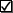Know that regression analysis concerns the form and direction of the relationship between variables; and is used for prediction.Know that correlation analysis concerns the strength of the relationship between variablesEnter data for an independent and a dependent variable using Microsoft Excel and generate a scatter diagram plot. Insert a trend line, regression equation and coefficient of determination on the plot.Generate a regression analysis, line fit plot, residual plot with prediction intervals, normal probability plot, and correlation coefficient in Microsoft Excel.Interpret regression coefficients, practical utility, statistical utility of the regression model; and interpret the correlation.Evaluate the assumptions of a regression.Use regression model for prediction.
 Module Notes The following sub modules contain summary notes for the two content topic areas of Module 2.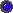Module 2.1: Simple Linear Regression: Present and Describe Linear RelationshipsModule 2.2: Test Linear Relationships and Make Predictions Assignment Select a realistic and interesting data set, consisting of a sample of approximately 50 observations. Your data set should include a quantitative (numerical) dependent or response variable (Y), and a quantitative independent or explanatory variable (X). Example: Y = Salary of USF Professor X = Years of Experience Example: Y = Weekly Closing Stock Price X = Weekly Closing SP500 1. Enter the X, Y data on Microsoft Excel Spreadsheet. 2. Create a scatter diagram of Y on X. 3. Create a scatter diagram of estimated Y on X;and insert the regression trend line, the regression equation, and the coefficient of determination (R Squared). 4. Generate the correlation coefficient. 5. Generate the numerical regression analysis, to include the line fit plot of estimated Y on X, the residual plot, and the normal probability plot. 6. Insert 95% prediction intervals on the line fit plot. 7. Be able to demonstrate your knowledge of the learning objectives, as applied to this Assignment, in Exam 2. 8. (Optional) Send Microsoft Excel file, with items 1 - 6, as an attachment in an e-mail to the instructor if you wish the instructor to review/give feedback on your work. This should be done not later than October 11, 2001 if you wish to receive instructor feedback. You may send parts of the assignment as you finish them, if you wish. 9. Notify the instructor, via e-mail, when you have completed Assignment 2, and wish to take Exam 2. Include Assignment 2 as an attachment to your e-mail if you did not do Item 8, above. This needs to be done BEFORE October 13, 2001. E-mail or fax completed Exam 2 back to the instructor NOT LATER THAN October 13, 2001. Optional Text Reading Anderson, D., Sweeney, D., & Williams, T. (2001). Contemporary Business Statistics with Microsoft Excel. Cincinnati, OH: South-Western. Chapter 3 (Section 3.5) Chapter 12 (Through Section 12.8)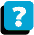About the Course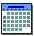Module Schedule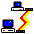WebBoard